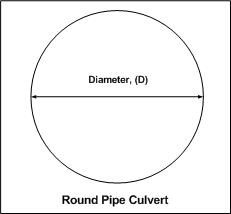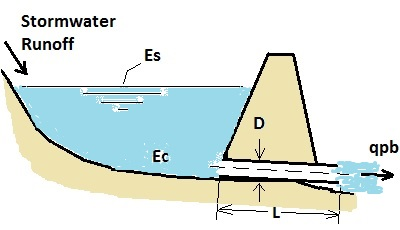* Log In to use the Calculate function * Become a Member!

Calculate the Discharge of a Pipe Outlet Control:

Calculation:
Designer/Checker:

Input:Water surface elevation at design volume (Es - ft or m)
Pipe centerline elevation (Ec - ft or m)
Trial diameter of pipe (D - in or mm)
Manning's roughness coefficient (n)
Length of pipe (L - ft or m)Output:

##### Login to enable the Formulas!

Kp =
C =
Discharge, Q =

 Start Using The Calculations >>> Become A Member Now!